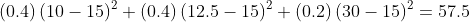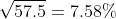Scan QR code or get instant email to install app

Question:

# An analyst estimates a stock has a 40% probability of earning a 10% return, a 40% probability of earning a 12.5% return, and a 20% probability of earning a 30% return. The stock’s standard deviation of returns based on this returns model is closest to:

A 7.58%.
explanation

1212Expected value = (0.4)(10%) + (0.4)(12.5%) + (0.2)(30%) = 15%
Variance =Standard deviation =